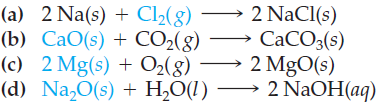×
Get Full Access to Introductory Chemistry - 5 Edition - Chapter 7 - Problem 33p
Get Full Access to Introductory Chemistry - 5 Edition - Chapter 7 - Problem 33p

×

# Consider the unbalanced chemical equation. A chemistry student tries to balance theISBN: 9780321910295 34

## Solution for problem 33P Chapter 7

Introductory Chemistry | 5th Edition

• Textbook Solutions
• 2901 Step-by-step solutions solved by professors and subject experts
• Get 24/7 help from StudySoup virtual teaching assistantsIntroductory Chemistry | 5th Edition

4 5 1 266 Reviews
18
4
Problem 33P

For each of the reactions, calculate how many grams of the product form when 2.4 g of the reactant in color completely reacts. Assume there is more than enough of the other reactant.Step-by-Step Solution:
Step 1 of 3

Solution 33P

Given chemical equation :The number of subscripts for each element in a chemical formula is like a form of spelling. Each chemical formula has its own unique set of elements and subscripts.

when balancing the chemical equation, You can’t change the subscripts, as changing the subscripts changes the substances themselves. Only the coefficients which describes the Moles of element in the reaction can be changed.

The correct balanced equation is :

2H2O → 2H2(g) + O2(g)

Step 2 of 3

Step 3 of 3

##### ISBN: 9780321910295

Introductory Chemistry was written by and is associated to the ISBN: 9780321910295. This textbook survival guide was created for the textbook: Introductory Chemistry, edition: 5. The full step-by-step solution to problem: 33P from chapter: 7 was answered by , our top Chemistry solution expert on 05/06/17, 06:45PM. Since the solution to 33P from 7 chapter was answered, more than 487 students have viewed the full step-by-step answer. This full solution covers the following key subjects: equation, correct, atom, chemical, Chemistry. This expansive textbook survival guide covers 19 chapters, and 2046 solutions. The answer to “?For each of the reactions, calculate how many grams of the product form when 2.4 g of the reactant in color completely reacts. Assume there is more than enough of the other reactant.” is broken down into a number of easy to follow steps, and 33 words.

Unlock Textbook Solution